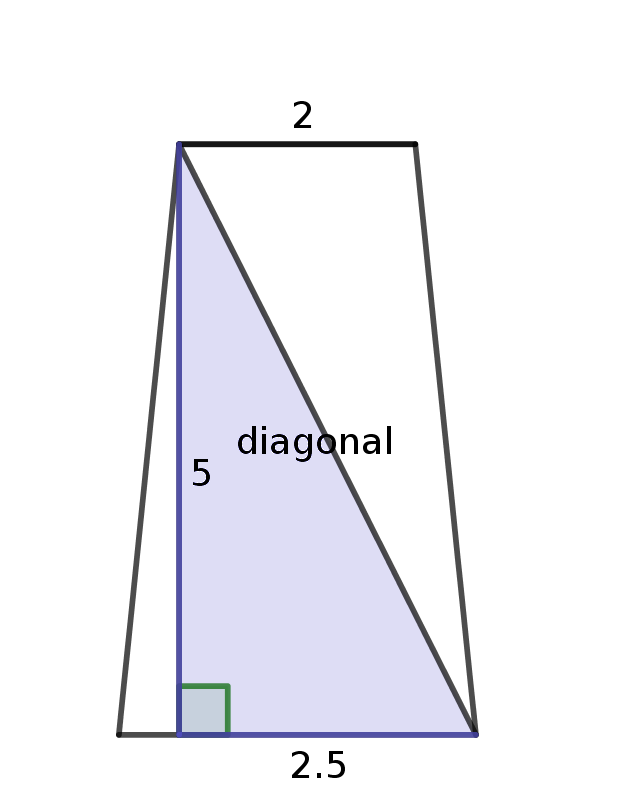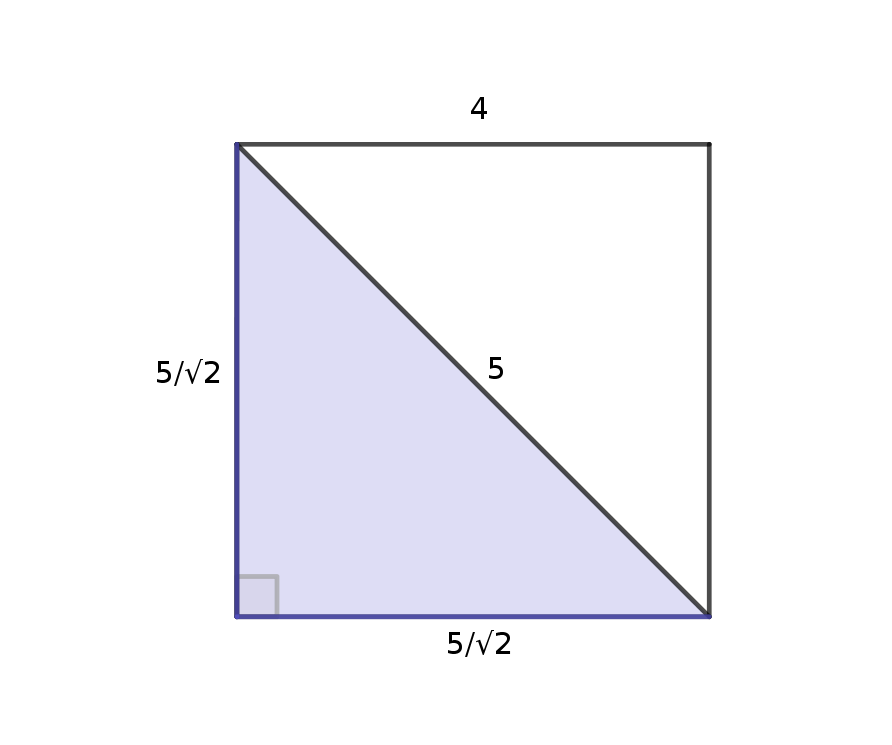# So do all isosceles trapezoids with 12 as the diagonal have area of 36 sqrt 3? What about vice versa?

• This post is deleted!

• [Originally posted in the Discussions]

So do all isosceles trapezoids with 12 as the diagonal have area of 36 sqrt 3? What about vice versa?

• You are right; since we saw in the video that the area of the trapezoid can be expressed just in terms of the length of its diagonal, then all trapezoids with a diagonal of length 12 will have the same area!

It's a really interesting question to consider the reverse line of logic. If two trapezoids have the same area, then do they have the same diagonal? Here let's look at a counterexample (an example that shows why this fact cannot be true):

The first trapezoid has bases of lengths 2 and 3, and a height of 5.The segment labeled 2.5 runs from the base of the altitude to the bottom right corner.

The area of this trapezoid is

$$\frac{1}{2} (2 + 3) \times 5 = \frac{25}{2}$$

Let's consider another trapezoid with the same area whose bases are almost equal in length, with height the same length as the bases. It would look almost like a square:The diagonal of the square is equal to 5. However, the diagonal of the first trapezoid must be larger than 5, because one of the legs is already equal to 5, and the Pythagorean Theorem tells us that

$$a^2 + b^2 = c^2$$

where c is the hypotenuse. These two trapezoids have the same area but different length diagonals, so this shows that not all isosceles trapezoids with the same area have the same length diagonals.

Happy Learning!

The Daily Challenge Team